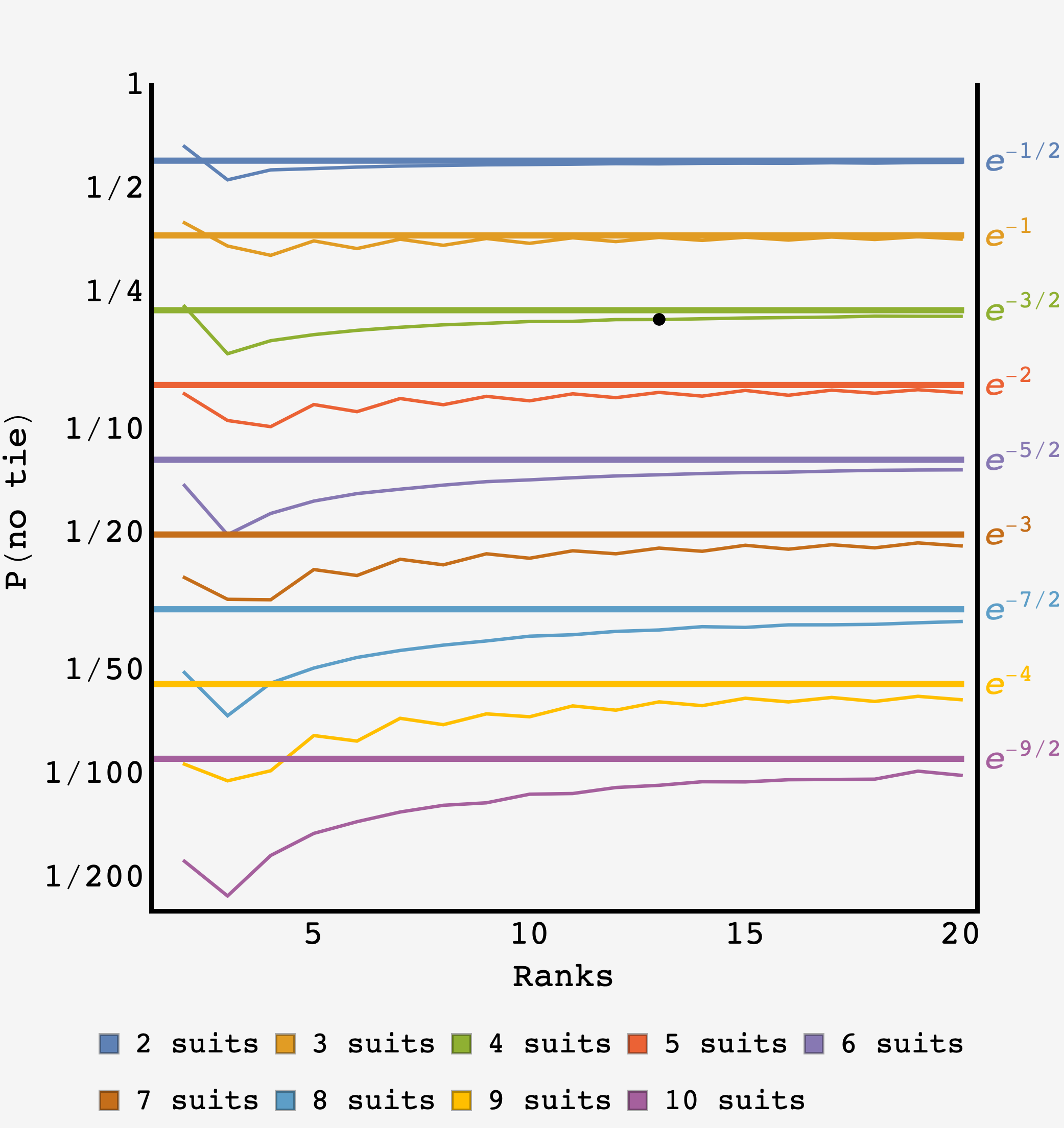Question: your friend Duane’s friend’s no-good, duplicitous, self-promoting grandchild has made extraordinary claims regarding their performance in the well-known cabin and road trip cardgame known as War. According to them, they’ve triumphed in a round where they won every single matchup with no ties, ending the game in a mere $26$ hands. Never one to let the feats of grandchildren go unchallenged, you set out to compute the probability of this occurrence. About how many rounds of War would one need to play to bear witness to their flawless victory?

## Solution

This problem has two pieces, one is the probability that the shuffle is such that every pair of cards has an unambiguous outcome, and the second is that every one of these matchups is won by your friend Duane’s friend’s grandchild. So,

$P(\text{rout}) = P(\text{rout}|\text{no ties})\times P(\text{no ties}).$

The second part is easy, given that we have an outcome with no ties, the probability that your friend Duane’s friend’s grandchild wins every one is just $1/2^{26},$ or, in the general case where there are $4r$ cards ($4$ each of $r$ kinds, where $r=13$ for the standard deck),

$P(\text{rout}|\text{no ties}) = \dfrac{1}{2^{2r}}.$

### No ties

As near as I can tell, there is no way to go straight for the probability of zero ties, $P(\text{no ties})$ and getting an exact answer calls for detailed accounting and combinatorics. However, it is possible to get the asymptotic result with a lot less effort. Also, the asymptotic result might be accurate surprisingly early on in the $r\rightarrow\infty$ path.

### Infinite ranks

We can build the shuffled War deck one pair at a time, working with $4r$ total cards in the limit where the number of ranks, $r,$ is large. For each pair, the first card is drawn uniformly and the second card needs to be one of the $4r - 3$ remaining cards that don’t have the same rank. The probability of this happening is

$P(\text{no tie in first pair}) = \frac{4r-3}{4r} = 1 - \frac{3}{4r}$

If we were dealing with small $r$ then we’d need to consider the possibility that subsequent pairs involve a rank that’s already been paired. However, the probability of this happening when $r$ is large is small compared to the prospect of forming a tie with an unused rank. So, the probability for a tie in the second pair is

$P(\text{no tie in second pair}) \approx 1 - \frac{3}{4r}$

as well (since $4r \gg 2$). Each time we pick new cards, we are much more likely to pick cards from an unused rank than to revisit an existing one.

As there are $2r$ pairs, the overall probability of no ties is

\begin{align} P(\text{no ties}) &\approx \left(1-\frac{3}{4r}\right)^{2r} \\ &= \left(1 - \frac{3/2}{2r}\right)^{2r} \end{align}

which, when we take $\lim\limits_{r\rightarrow\infty},$ is the definitional form for $e^{-3/2}.$

Putting it all together, the probability that your friend Duane’s friend’s grandchild wins their War match in a $2r=26$ hand rout is approximately

$P(\text{rout}) = P(\text{rout}|\text{no ties})\times P(\text{no ties}) = \frac{1}{2^{2r}}e^{-3/2}$

which is approximately $3.32489848\times 10^{-9}.$

### Expected waiting time

Answering the original question, we should expect to wait $T = P(\text{rout})^{-1}$ games to bear witness to the rout, or roughly $300,000,000$ games.

### Generalizing

In general, we can have $r$ ranks and $s$ suits. For a general deck, the number of hands played is $s\times r/2,$ the probability $\left(1-\frac{3}{2}\frac{1}{2r}\right)$ becomes $\left(1-\frac{s-1}{2}\frac{1}{sr/2}\right)$ and we have

$\boxed{P(\text{rout}) = \dfrac{1}{2^{sr/2}}e^{-(s-1)/2}}$

We can compare the asymptotic prediction for $P(\text{no tie})$ to a computer simulation for different values of $s$ and $r$.

When there are many different suits $s,$ we should expect to need many different ranks $r$ for a good prediction. This is because the asymptotic model assumes that the density of any given rank will be low. As the black dot shows, the asymptotic result is already quite good for the standard deck, accurate to within $\lt 6\%.$Comparison of asymptotic prediction (thick lines) and empirical results (thin lines, $N=1,000,000$ points per condition) for $P(\text{no tie})$ for different values of $s$ and $r.$ The black dot indicates the result for a standard deck of cards.

Here’s the Mathematica code used to generate the data above:

testDeck[ranks_, suits_] := (
tempDeck = Table[i~Mod~ranks + 1, {i, 1, suits ranks}];
tempDeck = RandomSample[tempDeck, suits ranks];
paired = Partition[tempDeck, 2];
Return[
If[AllTrue[paired, #[] != #[] &], 1, 0]
];)

testNDecks[ranks_, suits_, NN_] := {ranks,
Mean@ParallelTable[testDeck[ranks, suits], {i, 1, NN}]};

allData = Monitor[
Table[
Table[
testNDecks[ranks, suits, 1000000],
{ranks, 2, 20}
],
{suits, 2, 10}
],
{suits, ranks}
]